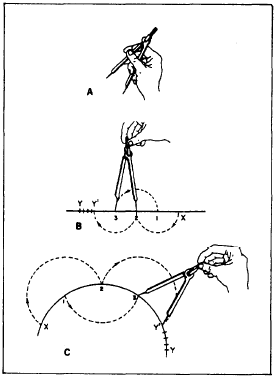Custom SearchUse of drafting templatesBasic EngineeringUse of the drafting scaleUSE OF THE DIVIDERS

As we stated in chapter 2, dividers are used to transfer measurements, to step off a series of equal distances, and to divide lines into a number of equal parts. Dividers are manipulated with one hand. In setting dividers (fig. 3-12, view A), holdFigure 3-12.-Use of the dividers.

one leg between the thumb and the first and second fingers, and hold the other leg between the third and fourth fingers. Place the second and third fingers on the inside of the legs; the dividers are opened by spreading these fingers apart. Dividers are closed by squeezing the thumb and first finger toward the fourth finger while gradually slipping out the other two fingers. To transfer measurements on a drawing, set the dividers to the correct distance, then transfer the measurements to the drawing by pricking the drawing surface very lightly with the points of the dividers.

To measure off a series of equal distances on the line, set the dividers to the given distance. Then step off this distance as many times as desired by swinging the dividers from one leg to the other along the line, first swinging clockwise 180 degrees, then counterclockwise 180 degrees, and so on.

In dividing either a straight line (fig. 3-12, view B) or a curved line (fig. 3-12, view C) into a given number of equal parts (for example, four) by trial, open the dividers to a rough approximation of the first division (in this case, one quarter of the line length) and step off the distance lightly, holding the dividers by the handle and pivoting the instrument on alternate sides of the line at each step. If the dividers fall short of the end of the line after the fourth step, hold the back leg in place and advance the forward leg, by guess, one quarter of the remaining distance. Repeat the procedure until the last step falls at the end of the line. Be careful during this process not to punch holes in the paper, but just barely mark the surface for future reference. To identify prick marks made with small dividers for future reference, circle the marks lightly with a pencil.Integrated Publishing, Inc. - A (SDVOSB) Service Disabled Veteran Owned Small Business